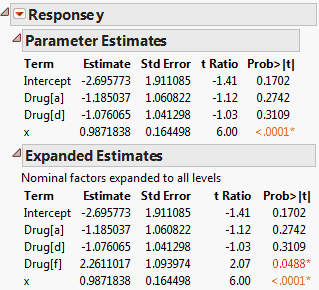Publication date: 11/10/2021

## Expanded Estimates

In dealing with parameter estimates, you must understand how JMP codes nominal and ordinal columns. For more information about how nominal columns are coded, see Details of Custom Test Example. For more information about how ordinal columns are coded and modeled, see Nominal Factors and Ordinal Factors.

Use the Expanded Estimates option when there are nominal terms in the model and you want to see details for the full set of estimates. The Expanded Estimates option provides the estimates, their standard errors, t ratios, and p-values.

#### Example of an Expanded Estimates Report

1. Select Help > Sample Data Library and open Drug.jmp.

2. Select Analyze > Fit Model.

3. Select y and click Y.

4. Select Drug and x, and then click Add.

5. Click Run.

6. Click the Response y red triangle and select Estimates > Expanded Estimates.

The Expanded Estimates report, along with the Parameter Estimates report, is shown in Figure 3.23. Note that an estimate for the term Drug[f] appears in the Expanded Estimates report. The null hypothesis for the test is that the mean for the Drug f group does not differ from the overall mean. The test for Drug[f] is significant at the 0.05 level, suggesting that the mean response for the Drug f group differs from the overall response. See Interpretation of Tests for Expanded Estimates.

Figure 3.23 Comparison of Parameter Estimates and Expanded Estimates### Interpretation of Tests for Expanded Estimates

Suppose that your model consists of a single nominal factor that has n levels. That factor is represented by n-1 indicator variables, one for each of n-1 levels. The parameter estimate corresponding to any one of these n-1 indicator variables is the difference between the mean response for that level and the average response across all levels. This representation is due to how JMP codes nominal variables. See Details of Custom Test Example. The parameter estimate is often interpreted as the effect of that level.

For example, in the Cholesterol.jmp sample data table, consider the single factor treatment and the response June PM. The parameter estimate associated with the term, or indicator variable, treatment[A] is the difference between the mean of June PM for treatment A and the overall mean of June PM.

The effects across all levels of a nominal variable are constrained to sum to zero. Consider the effect of the last level in the level ordering, namely, the level that is coded with –1s. The effect of this level is the negative of the sum of the effects across the other n-1 levels. It follows that the effect of the last level is the negative of the sum of the parameter estimates across the other n-1 levels.

The Expanded Estimates option in the Estimates menu calculates missing estimates, tests for all effects that involve nominal columns, and shows them in a text report. You can verify that the mean (or sum) of the estimates across the levels of any such effect is zero. In particular, this relationship indicates that these estimates, and their associated tests, are not independent of each other.

In the Drug.jmp report shown in Figure 3.23, the estimates for the terms associated with Drug are based on a model that includes the covariate x.

Notes:

The estimate for Drug[a] is the difference between the least squares mean for Drug a and the overall mean of y.

The estimate for Drug[f], given in the Expanded Estimates report, is the negative of the sum of the estimates for Drug[a] and Drug[d].

The t test for Drug [f] presented in the Expanded Estimates report tests whether the response for the Drug f group differs from the overall mean response.

If nominal factors are involved in high-degree interactions, the Expanded Estimates report can be lengthy. For example, a five-way interaction of two-level nominal factors produces only one parameter estimate but has 25 = 32 expanded effects, which are all identical up to sign changes.